## ASIC Timing Interview Questions

TIMING Interview QuestionsTiming, an important parameter associated with Sequential Circuit design will be discussed in this tutorial. We will begin with the general concepts associated with timing and then will proceed with examples to better understand their application to digital design. This tutorial consists of three sections.

PART 1 Introduction and terminology

PART 2 Equations

PART 3 Example problems

PART 1:  Introduction and terminology

========================================================================

A Digital System Design circuit can be characterized as a  'Combinational circuit' or a 'Sequential Circuit' and while calculating for Timing we will have to first identify what type of circuit is involved.

---------------------------------------------------------------------------------------------------------------------------

Q1.How do we know, if given a circuit, whether it is a Combinational Circuit or a Sequential Circuit?

[Ans] If a circuit has only combinational devices (e.g.. gates like AND, OR etc and MUX(s))and no Memory elements then it is a Combinational circuit. If the circuit has memory elements such as Flip Flops, Registers, Counters, or other state devices then it is a Sequential Circuit.  Synchronous sequential circuits will also have a clearly labeled clock input.

---------------------------------------------------------------------------------------------------------------------------

Q2. Are the following circuits combinational or sequential?

[Ans]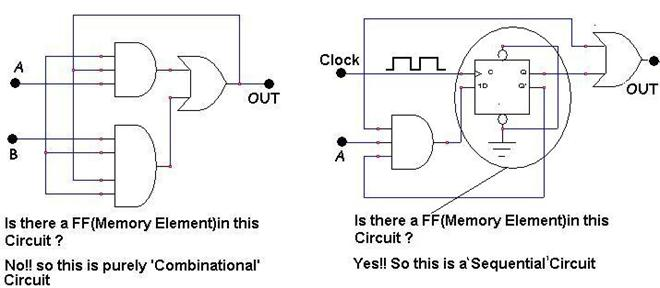---------------------------------------------------------------------------------------------------------------------------

Q3. Why do we have to identify the type of circuit? Does it really matter?

[Ans]  It is important to identify the type of circuit because our timing calculation approach differs accordingly. Combinational circuits timing analysis deals primarily with propagation delay issues. Sequential circuits have additional specific timing characteristics that must be satisfied in order to prevent metastability, including setup time, hold time, and minimum clock period. Designers of sequential devices must specify these important timing characteristics in order to allow the device to be used without error.

---------------------------------------------------------------------------------------------------------------------------

Q4. Do all Digital Devices like gates and Flip Flops have timing parameters?

[Ans] Yes, all digital devices have timing parameters. In the real environment (not Ideal as in our lab) there will be a real (non zero) value associated with every digital device. Observe the examples below

Example 1 and 2:---------------------------------------------------------------------------------------------------------------------------

Q5.Phew!!! So many things all at the same time.....what is propagation delay?

[Ans] All devices have some delay associated with transferring an input change to the output. These changes are not immediate in a real environment. This delay that is due to the signal propagation through the device is called the propagation delay

---------------------------------------------------------------------------------------------------------------------------

Q6. What is Setup time?

[Ans] Setup time is a timing parameter associated with Sequential Devices (for simplicity henceforth I will be only referring to the Flip Flop). The Setup time is used to meet the minimum pulse width requirement for the first (Master) latch makes up a flip flop is. More simply, the setup time is the amount of time that an input signal (to the device) must be stable (unchanging) before the clock ticks in order to guarantee minimum pulse width and thus avoid possible metastability.

---------------------------------------------------------------------------------------------------------------------------

Q7. What is Hold time?

[Ans] Hold time is also a timing parameter associated with Flip Flops and all other sequential devices. The Hold time is used to further satisfy the minimum pulse width requirement for the first (Master) latch that makes up a flip flop. The input must not change until enough time has passed after the clock tick to guarantee the master latch is fully disabled. More simply, hold time is the amount of time that an input signal (to a sequential device) must be stable (unchanging) after the clock tick in order to guarantee minimum pulse width and thus avoid possible metastability.

---------------------------------------------------------------------------------------------------------------------------

Q8. Can you give an example that can help me better understand the Setup and Hold time concept?

[Ans] Lets consider the situation where-in I am the Flip Flop and I am to receive an Input (a photo of an old friend whom I have to recognize ) now the amount of time it would take to setup the photo in the right position so that it is visible to me from where I am sitting  (since I am lazy to walk over) can be considered as the "Setup time". Now once shown the photo the amount of time that I keep staring at it till I feel comfortable enough to start relating it to known faces can be considered as the "Hold time".

---------------------------------------------------------------------------------------------------------------------------

Q9. What is a timing diagram? Can we use it to better understand Setup and Hold time?

[Ans] Timing diagram is a complete description of a digital machine. We can use the timing diagram (waveform) to illustrate Setup and Hold time. Observe the waveform given below: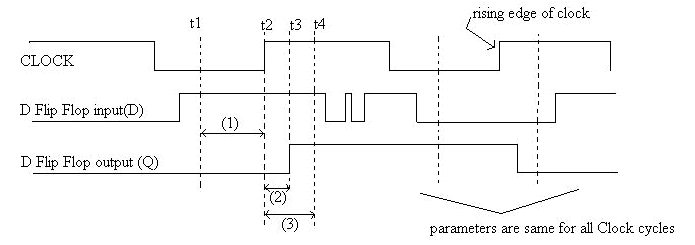From the timing diagram we observe that we have three signals: the Clock, the Flip Flop Input (D) and the Flip Flop output (Q). We have four timing instances and three time periods. The inferences from this waveform will help us understand the concept of propagation delay Setup and Hold time.

(1) i.e. [t2 - t1] is the Setup Time: the minimum amount of time Input must be held constant BEFORE the clock tick. Note that D is actually held constant for somewhat longer than the minimum amount. The extra “constant” time is sometimes called the setup margin.

(2) i.e. [t3 - t2] is the Propagation delay of the Flip Flop: the minimum/maximum time for the input to propagate and influence the output.

(3) i.e. [t4 - t2] is the Hold time: the minimum amount of time the Input is held constant AFTER the clock tick. Note that Q is actually held constant for somewhat longer than the minimum amount. The extra “constant” time is sometimes called the hold margin.

(The above timing diagram has 2 clock cycles; the timing parameters for the second cycle will also be similar to that of the first cycle)

---------------------------------------------------------------------------------------------------------------------------

PART 2: Equations

========================================================================

This part of the tutorial introduces us to the various different timing calculations associated with this course. We may be given a sequential circuit and asked to solve for the timing parameters. Let us discuss in detail how we should approach such problems.

Q11. What is the first thing to do if given a sequential circuit and asked to analyze its timing?

[Ans] Given a sequential circuit it is often advisable to first divide the circuit in to three distinct parts i.e. Input Logic, State Memory and the Output Logic. Such division will also help with identifying whether the given circuit is Mealy or Moore.  The input logic (Next State Logic) and the output logic blocks constitute of only combinational logic components like gates, muxes etc. The state memory block is made of only sequential components like Flip Flops etc.

---------------------------------------------------------------------------------------------------------------------------

Q12. Can you explain the answer to Q11 more elaborately?

[Ans] Let me explain using block diagrams. A given sequential circuit can be represented in either of the two ways as shown below.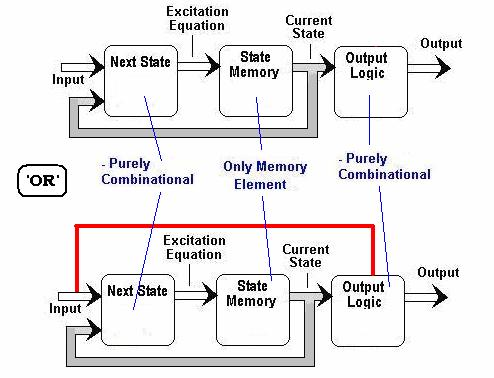The first representation shows the sequential circuit where the input(s) have to pass through the State memory to affect the output. Such machines are called Moore machines.

The second representation shows the ‘red bypass’ which signifies that the output can be directly affected by the inputs without having to pass through the state memory device(s). Such devices are called Mealy machines.

---------------------------------------------------------------------------------------------------------------------------

Q13. Can you explain this with an example?

[Ans] Ok, consider the sequential circuit shown belowLet us now identify the three distinct parts in this given sequential circuit. Observe the division on the circuit below.

.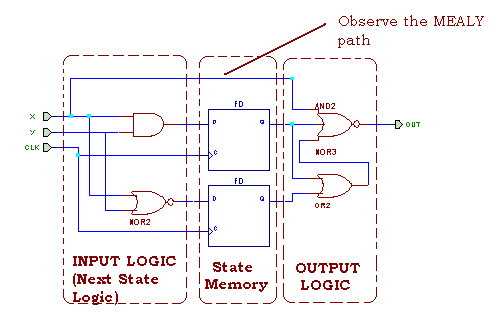Observation: This given circuit is a MEALY machine.

---------------------------------------------------------------------------------------------------------------------------

Q14. Now that we have divided the circuit into more distinct parts how do we proceed with calculating the timing parameters?

[Ans] Remember from our discussion in Part 1 of this tutorial we know that combinational devices and sequential devices have different timing parameters. Now that we have separated them both into separate blocks we can define them more clearly. To relate them to the blocks let us follow some convention (already discussed in part 1). Let us refer to the timing parameters for the input logic (also referred to as the next state logic) and output logic with the letter ‘F’ and ‘G’ respectively. Similarly, let us refer to all timing parameters associated with the State memory block with the letter ‘R’.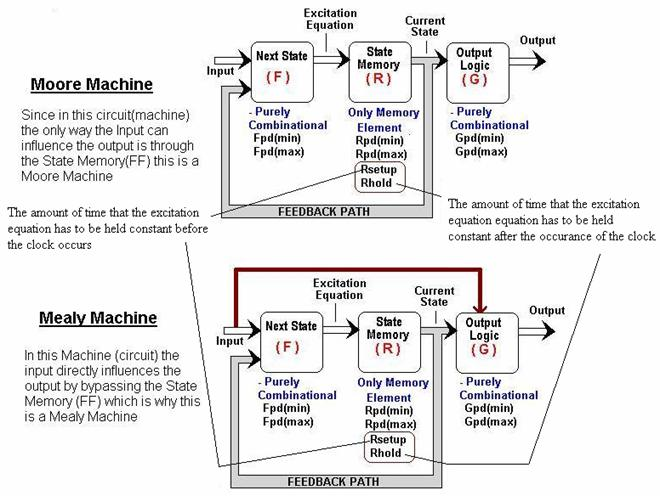---------------------------------------------------------------------------------------------------------------------------

Q15. What timing parameters are commonly used?

[Ans] The list of the timing parameters that you may be asked to calculate for a given sequential circuit is

1.      Propagation delay, Clock to Output (minimum)

2.      Propagation delay, Clock to Output (maximum)

3.      Propagation delay, Input to Output (minimum)

4.      Propagation delay, Input to Output (maximum)

5.      Setup Time (Data input before clock)

6.      Hold Time (Data input after clock)

7.      Maximum Clock rate (or its reciprocal, minimum clock period)

---------------------------------------------------------------------------------------------------------------------------

Q16. How do we find the Propagation delay, Clock to Output?

[Ans] Propagation delay (PD) for the circuit can be calculated as the summation of all delays encountered from where the clock occurs to the output. In short, the delays of the State memory and the output logic.

PD Clock- Output (min) = Rpd (min) + Gpd (min)

PD Clock- Output (max) = Rpd (max) + Gpd (max)

---------------------------------------------------------------------------------------------------------------------------

Q17. How do we find the Propagation delay, Input to Output?

[Ans] This is a property associated with Mealy machines only. In other words, for a Moore machine the value for this timing parameter is infinity (∞). The calculation (for mealy machines) is the summation of all propagation delays encountered between the input (that influences the output by bypassing the state memory) and the output.

For MOORE machines:

PD Input- Output (min) = infinity (∞)

PD Input- Output (max) = infinity (∞)

For MEALY Machines

PD Input- Output (min) = Gpd (min)

PD Input- Output (max) = Gpd (max)

---------------------------------------------------------------------------------------------------------------------------

Q18. How do we calculate Setup time?

[Ans] The calculation for setup time is the sum of the setup time for the concerned flip flop and the maximum delay from the input logic.

T SETUP = RSETUP+ Fpd (MAX)

---------------------------------------------------------------------------------------------------------------------------

Q19. How do we get the value for the Hold time?

[Ans] The value for the Hold time can be obtained by the following formulae

T HOLD = RHOLD - Fpd (MIN)

The concern here is how soon (minimum time) an erroneous input can propagate in from the Input logic while the Flip Flop is attempting to hold on to a stable value. The negative sign can be associated with ‘after the clock occurs’ to ease in remembering this formulae.

---------------------------------------------------------------------------------------------------------------------------

Q20. How do we calculate the Maximum Clock rate (MCLK)?

[Ans] Maximum clock rate is calculated using the formula

MCLK = 1/ TMIN

So we will have to calculate TMIN first. TMIN here refers to the minimum time period for correct operation of the circuit, so it is calculated using all worst cases (maximum delays).

TMIN =   Fpd (MAX) + RSETUP + Rpd (MAX)

So having found the minimum clock period let us now calculate for the MCLK

MCLK = 1/ TMIN   = (Fpd (MAX)   + RSETUP + Rpd (MAX) )-1

---------------------------------------------------------------------------------------------------------------------------

[Ans] Ok, here is everything we discussed so far in Part 2

1.    PD Clock- Output (min) = Rpd (min) + Gpd (min)

2.    PD Clock- Output (max) = Rpd (max) + Gpd (max)

3.    PD Input- Output (min) = infinity (∞) (For MOORE machines)

4.    PD Input- Output (max) = infinity (∞) (For MOORE machines)

5.    PD Input- Output (min) = Gpd (min) (For MEALY machines)

6.    PD Input- Output (max) = Gpd (max) (For MEALY machines)

7.    T SETUP = RSETUP+ Fpd (MAX)

8.    T HOLD = RHOLD - Fpd (MIN)

9.    MCLK = 1/ TMIN   = (Fpd (MAX)   + RSETUP + Rpd (MAX) )-1

---------------------------------------------------------------------------------------------------------------------------

PART 3:  Examples

========================================================================

Q23. Can we go through a timing example (solved problem) so that we can have a better understanding of the concepts dealt so far?

[Ans] Sure, here is a simple example to begin with, you are given a sequential circuit as shown below and asked to calculate all the timing parameters discussed in Part 2 of this tutorial. The information provided to you with the question is the individual timing parameters of the components listed in the table below.

 Device Propagation Delay (Minimum) Propagation Delay (Maximum) Setup Time Hold Time D Flip Flop 4 ns 8 ns 10 ns 3 ns NAND Gate 3 ns 6 ns X X Bubbled AND Gate 2 ns 4 ns X XWith this information we can approach the problem as discussed in Part 2 of this tutorial i.e. we shall first divide the given circuit into three distinct parts and then solve for timing. With practice, we can afford to skip this step of dividing the circuit into distinct parts (thereby saving time) and directly solve for timing. Since this is the first example I shall religiously follow the steps discussed in Part 2.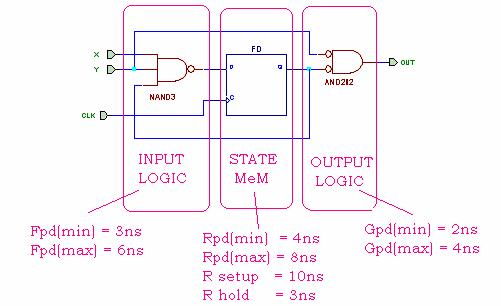Observation: This is a MEALY Machine.

Now let us calculate for all the timing parameters.

1.      PD Clock- Output (min) = Rpd (min) + Gpd (min)   = 4ns + 2ns = 6ns

2.      PD Clock- Output (max) = Rpd (max) + Gpd (max)  = 8ns + 4ns = 12ns

3.      PD Input- Output (min) = Gpd (min)  = 2ns

4.      PD Input- Output (max) = Gpd (max)  = 4ns

5.      T SETUP = RSETUP+ Fpd (MAX)  = 10ns + 6ns = 16ns

6.      T HOLD = RHOLD - Fpd (MIN)   = 3ns – 3ns = 0ns.

7.      TMIN  = Fpd (MAX)   + RSETUP + Rpd (MAX)  = 6ns +10ns + 8ns = 24ns

8.      MCLK = 1/ TMIN   = (Fpd (MAX)   + RSETUP + Rpd (MAX) )-1    = 1/24ns.

---------------------------------------------------------------------------------------------------------------------------

Q24. Can we go through another timing example (solved problem) using more than one Flip Flop?

[Ans]  Ok, here is an example (notice how I write down the corresponding timing values for simplicity in understanding)

Given with the question is the individual timing parameter for all the components used in the Circuit. Observe the table given below.

 Device Propagation Delay (minimum) Propagation Delay (maximum) Setup Time Hold Time D Flip Flop 2ns 6ns 4ns 2ns AND Gate 2ns 4ns X X 2 i/p NOR Gate 2ns 3ns X X OR Gate 2ns 3ns X X 3 i/p NOR Gate 1ns 2ns X XWriting the timing parameters next to the components (for ease in solving)So with the timing parameters next to the components the circuit now looks like thisDividing the circuit into distinct parts is left to the reader (will give the reader some hands-on practice)

Now let us calculate for all the timing parameters.

1.      PD Clock- Output (min) = Rpd (min) + Gpd (min)   =  2ns + 1ns = 3ns

2.      PD Clock- Output (max) = Rpd (max) + Gpd (max)  = 6ns + 3ns + 2ns = 11ns

3.      PD Input- Output (min) = Gpd (min) (For MEALY machines)  = 1ns

4.      PD Input- Output (max) = Gpd (max) (For MEALY machines) = 2ns

5.      T SETUP = RSETUP+ Fpd (MAX)  = 4ns + 4ns = 8ns

6.      T HOLD = RHOLD - Fpd (MIN)   = 2ns – 2ns = 0ns.

7.      TMIN  = Fpd (MAX)   + RSETUP + Rpd (MAX)  = 3ns + 4ns + 4ns + 6ns = 17ns

8.      MCLK = 1/ TMIN   = (Fpd (MAX)   + RSETUP + Rpd (MAX) )-1    = 1/17ns.

---------------------------------------------------------------------------------------------------------------------------

Q25. Are these two solved examples enough to introduce us to the timing concepts necessary for this course?

[Ans] Absolutely, the two examples together cover almost all the concepts necessary to get you started with understanding timing problems (the intent of this tutorial). More examples would result in spoon-feeding and would not be recommended. Interested students can now read the text and attempt to solve other timing related questions for practice.

Verilog FAQ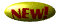Synthesis FAQ

Digital FAQ

Timing FAQ

ASIC FAQCmos FAQ

Misc FAQ

Home